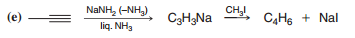Problem: Give structures for the products of each of the following reactions:

94% (53 ratings)
Problem Details

Give structures for the products of each of the following reactions:What scientific concept do you need to know in order to solve this problem?

Our tutors have indicated that to solve this problem you will need to apply the Alkynide Alkylation concept. You can view video lessons to learn Alkynide Alkylation Or if you need more Alkynide Alkylation practice, you can also practice Alkynide Alkylation practice problems .

What is the difficulty of this problem?

Our tutors rated the difficulty of Give structures for the products of each of the following re... as medium difficulty.

How long does this problem take to solve?

Our expert Organic tutor, Chris took 3 minutes to solve this problem. You can follow their steps in the video explanation above.

What textbook is this problem found in?

Our data indicates that this problem or a close variation was asked in . You can also practice practice problems .